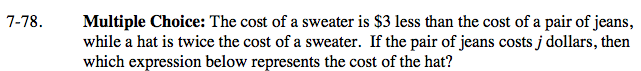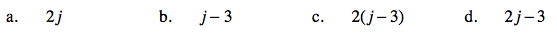### Home > AC > Chapter 7 > Lesson 7.2.3 > Problem7-78

7-78.
1. Multiple Choice: The cost of a sweater is \$3 less than the cost of a pair of jeans, while a hat is twice the cost of a sweater. If the pair of jeans costs j dollars, then which expression below represents the cost of the hat? Homework Help ✎

1. 2j

2. j − 3

3. 2(j − 3)

4. 2j − 3First find the cost of a sweater using the variable j.

Sweater = j − 3

Using that information, can you find the price of the hat?

Hat = 2(j − 3), (c)